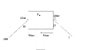# Using 0 Moment to find a force.

• weedannycool

#### weedannycool

how do you find a 0 moment on a system. for example if i had a block with two forces in tension either side and i know 1 tension value and i want to find the other which will cause zero moment at a point ( say O ). any ideas??

Thanks

__________

Daniel

i'll draw a pic. i want to find T so there is zero moment at O. but the block is suported

#### Attachments

•pic1.jpg
9.1 KB · Views: 463
Last edited:
hope you can understand that :/

Resolve the forces into components and sum the moments about point 0. You should end up with enough equations to solve for T.

CS

## 1. What is the 0 Moment method and how does it work?

The 0 Moment method is a technique used in engineering and physics to find the magnitude and direction of a force acting on an object. It involves taking the sum of all the moments (or torques) acting on an object and setting it equal to 0. This allows us to solve for the unknown force.

## 2. When is the 0 Moment method typically used?

The 0 Moment method is commonly used in situations where there are multiple forces acting on an object, and we need to determine the magnitude and direction of one of those forces.

## 3. Can the 0 Moment method be used for both static and dynamic systems?

Yes, the 0 Moment method can be used for both static and dynamic systems. However, in dynamic systems, the method may need to be modified to account for changing forces over time.

## 4. Are there any limitations to using the 0 Moment method?

One limitation of the 0 Moment method is that it assumes the object is in equilibrium, meaning that all forces and torques acting on it are balanced. It also assumes that the object is rigid and does not deform under the applied forces.

## 5. How accurate is the 0 Moment method in determining forces?

The accuracy of the 0 Moment method depends on the accuracy of the measurements and assumptions made about the system. If the measurements are precise and the assumptions are valid, the method can provide an accurate determination of the unknown force.